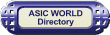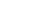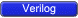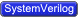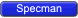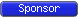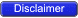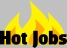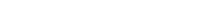Introduction One of the most common questions in interviews is how to calculate the depth of a FIFO. Fifo is used as buffering element or queueing element in the system, which is by common sense is required only when you slow at reading than the write operation. So size of the FIFO basically implies the amount of data required to buffer, which depends upon data rate at which data is written and the data rate at which data is read. Statistically, Data rate varies in the system majorily depending upon the load in the system. So to obtain safer FIFO size we need to consider the worst case scenario for the data transfer across the FIFO under consideration.For worst case scenario, Difference between the data rate between write and read should be maximum. Hence, for write operation maximum data rate should be considered and for read operation minimum data rate should be considered.So in the question itself, data rate of read operation is specified by the number of idle cycles and for write operation, maximum data rate should be considered with no idle cycle.So for write operation, we need to know Data rate = Number of data * rate of clock. Writing side is the source and reading side becomes sink, data rate of reading side depends upon the writing side data rate and its own reading rate which is Frd/Idle_cycle_rd.In order to know the data rate of write operation, we need to know Number of data in a Burst which we have assumed to be B.So following up with the equation as explained below: Fifo size = Size to be buffered = B - B * Frd / (Fwr* Idle_cycle _rd ).Here we have not considered the sychnronizing latency if Write and Read clocks are Asynchronous. Greater the Synchronizing latency, higher the FIFO size requirement to buffer more additional data written.Example : FIFO Depth CalculationAssume that we have to design a FIFO with following requirements and We want to calculate minumum FIFO depth,A synchronized fifo Writing clock 30MHz - F1 Reading clock 40MHz - F2 Writing Burst Size - B Case 1 : There is 1 idle clock cycle for reading side - I Case 2 : There is 10 idle clock cycle for reading side - IFIFO depth calculation = B - B *F2/(F1*I)If if we have alternate read cycles i.e between two read cycle there is IDLE cycle. FIFO depth calculation = B - B * F2/(F1*2)In our present problem FIFO depth = B - B *40/(30*2) = B(1-2/3) = B/3That means if our Burst amount of data is 10 , FIFO DEPTH = 10/3 = 3.333 = 4 (approximatly)If B = 20 FIFO depth = 20/3 = 6.6 = 7 or 8 (clocks are asynchronous)If B = 30 FIFO depth = 30/3 = 10 10+1 = 11 (clocks are asynchronous)If 10 IDLE cycles betweeen two read cycles . FIFO DEPTH = B - B *F2/(F1*10) . = B(1-4/30) = B * 26 /30